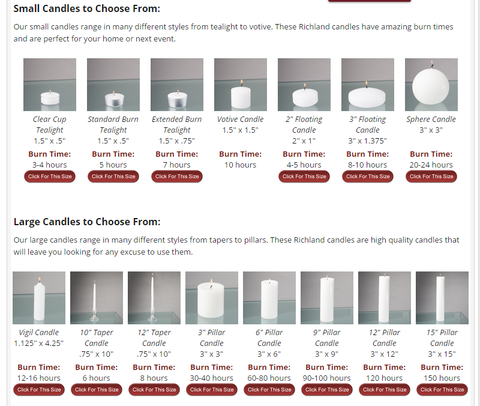# Candle Burn Times

How to calculate the burn time of your candle

Before you light the candle use a scale to weigh it. Once you have the original weight trim the wick and light the candle, letting it burn for four hours. When the four hours are up blow it out, let it cool, and weigh it again. Subtract the weight after the burn cycle from the original weight and divide by the number of hours. The number you end up with is your hourly burn rate.

Once you have the hourly burn rate divide the weight of the whole candle* by the hourly rate and you end up with the number of hours your candle will burn.

Amount Consumed (Original wt. - wt. After Burning) ÷ Hours Burnt = Hourly Burn RateOriginal wt. (minus wt. of container) ÷ Hourly Burn Rate = Approximate Burn Time

• If you are testing a container candle make sure to subtract the weight of the container from the weight of the whole candle before dividing by your hourly rate.
• Ounces work fine for calculating the burn rate but if your scales has grams as an option we would recommend using that. Since grams are so much smaller than ounces you end up with nicer numbers ( 5 grams/hr instead of .16 ounces/hr. ).
• Although one burn cycle (four hours) will give you a fairly accurate hourly burn rate numerous cycles (three or four) will produce a much more accurate number. The first burn cycle is always a little off because the wick is not yet completely saturated with wax, color, and fragrance.